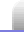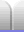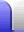# Nicomachus' Theorem and Number Theory

I stumbled across an interesting mathematical observation (while looking for info about a particular old maths problem being solved).

13 + 23 = 32

I decided to explore this pattern to see if it continued, or how easy it was to predict. Was it simply the sum of consecutive cubic numbers adding up to the next square number? No.

13 + 23 + 33 = 36 = 62
13 + 23 + 33 + 43 = 100 = 102
13 + 23 + 33 + 43 + 5 3 = 225 = 152

The numbers on the right hand side are the squares of the triangular numbers, which are the sum of the first n numbers. So the sum of the first n cubic numbers is the square of the sum of the first n numbers. This is quite interesting for me because I quite like the sequence of triangular numbers.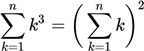I wondered what this is called, and a surprisingly quick search turned up Faulhaber's formula. The relationship is not itself Faulhaber's formula, but is a particular example of that formula. Expanded to remove the sum function, this particular example probably looks a bit more familiar for several of my students.Named after Johann Faulhaber, a late 16th/early 17th century German mathematician, Faulhaber's formula is a generalised method of adding any sum of consecutive numbers which have been raised to some arbitrary power. I'm not going to delve into it in any depth here.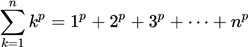The equation I found has been known of for much longer, and is sometimes called Nicomachus' Theorem (more generally, squared triangular number), after Nicomachus of Gerasa, a Pythagorean who lived about 100 years after Christ. He commented on the relationship in his book Introduction to Arithmetic, which is his only surviving work, but didn't fully explore the relationship.

So what I stumbled upon is nothing new but it has proved inspiring for the last two thousand years. The Wikipedia listing squared triangular number says: Stroeker (1995) claims that "every student of number theory surely must have marveled at this miraculous fact". Number theory is the branch of mathematics which deals mainly with integers.

"Mathematics is the queen of the sciences –
and number theory is the queen of mathematics."
Carl Friedrich Gauss (1777–1855)Home Astronomy Chemistry Electronics & Computers Mathematics Physics Field Trips Turn on javascript for email link